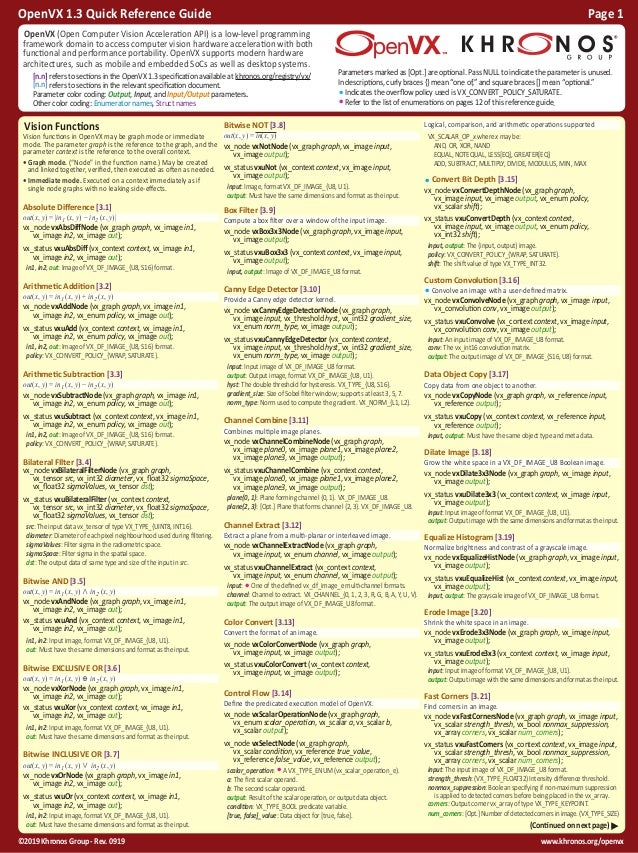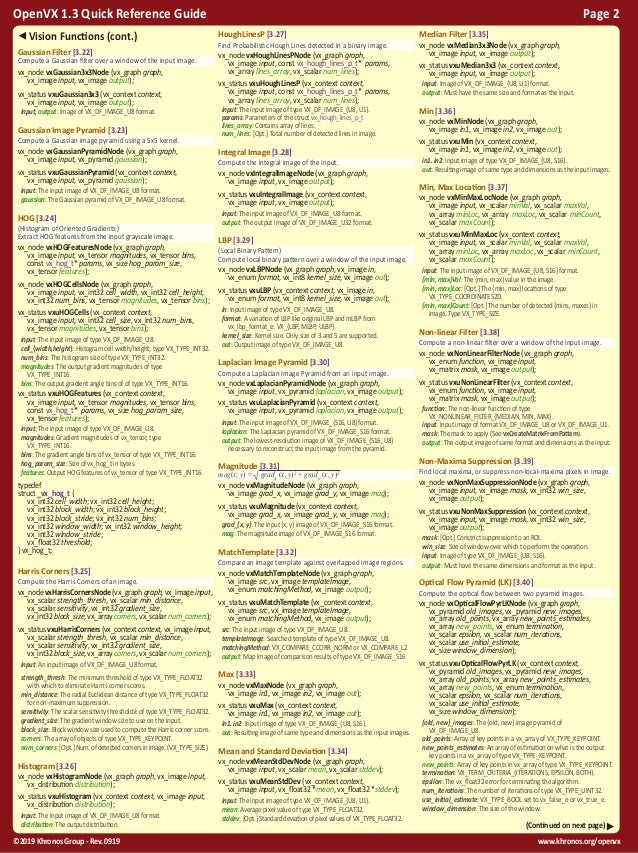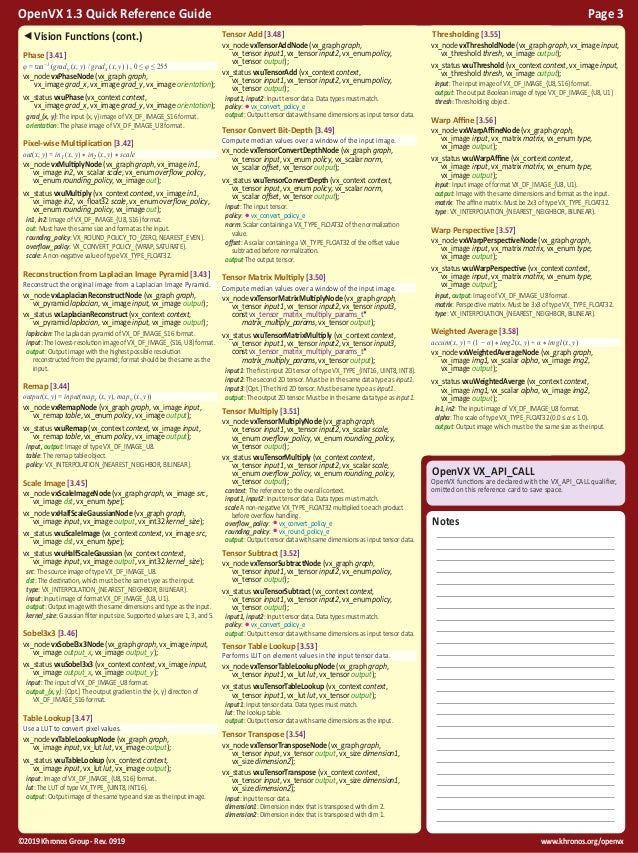Successfully reported this slideshow.Upcoming SlideShare
×

ofUpcoming SlideShare
Next

0 Likes

Share

# OpenVX 1.3 Reference Guide

The Khronos Group Official OpenVX 1.3 Reference Guide

See all

### Related Audiobooks

#### Free with a 30 day trial from Scribd

See all
• Be the first to like this

### OpenVX 1.3 Reference Guide

10. 10. www.khronos.org/openvx©2019 Khronos Group - Rev. 0919 OpenVX 1.3 Quick Reference Guide Page 10 Neural Network Extension This extension enables execution and integration of deep neural networks in OpenVX processing graphs. The extension works with the vx tensor object, which is a multidimensional array with an arbitrary number of dimensions and can represent all varieties of data typically used in a deep neural network. This extention is documented in The OpenVX Neural Network Extension available in the OpenVX registry at khronos.org/ registry/OpenVX/ Kernel Names When using vxGetKernelByName with this extension, the following strings are used in the name parameter to specify the neural networks extension kernel names. org.khronos.nn_extension.convolution_layer org.khronos.nn_extension.fully connected_layer org.khronos.nn_extension.pooling_layer org.khronos.nn_extension.softmax_layer org.khronos.nn_extension.local_response_normalization_layer org.khronos.nn_extension.activation_layer org.khronos.nn_extension.roi pooling_layer org.khronos.nn_extension.deconvolution_layer Data Structures Input parameters for a convolution operation. struct _vx_nn_convolution_params_t (vx_size) padding_x/y: The number of elements added at each side in the x/y dimension of the input. (vx_enum) overflow_policy: •vx_convert_policy_e (vx_enum) rounding_policy: •vx_round_policy_e (vx_enum) down_scale_size_rounding: Rounding method for calculating output dimensions from vx_nn_rounding_type_e listed in Enumerators below. (vx_size) dilation_x/y: Inflate the kernel by inserting zeros between the kernel elements in the x/y direction. Input parameters for a deconvolution operation. struct _vx_nn_deconvolution_params_t (vx_uint32) padding_x/y: The number of elements added at each side in the x/y dimension of the input. (vx_enum) overflow_policy: •vx_convert_policy_e (vx_enum) rounding_policy: •vx_round_policy_e (vx_size) a_x/y: A user-specified quantity used to distinguish between the upscalex/y different possible output sizes. Enumerators The following enumerators are enabled with this extension. vx_kernel_nn_ext_e: VX_KERNEL_CONVOLUTION_LAYER VX_KERNEL_FULLY_CONNECTED_LAYER VX_KERNEL_POOLING_LAYER VX_KERNEL_SOFTMAX_LAYER VX_KERNEL_NORMALIZATION_LAYER VX_KERNEL_ACTIVATION_LAYER VX_KERNEL_ROI_POOLING_LAYER VX_KERNEL_DECONVOLUTION_LAYER VX_KERNEL_LOCAL_RESPONSE_NORMALIZATION_LAYER vx_nn_activation_function_e: VX_NN_ACTIVATION_LOGISTIC VX_NN_ACTIVATION_HYPERBOLIC_TAN VX_NN_ACTIVATION_{RELU, BRELU, SOFTRELU} VX_NN_ACTIVATION_{ABS, SQUARE, SQRT, LINEAR} vx_nn_enum_e: VX_ENUM_NN_ROUNDING_TYPE VX_ENUM_NN_POOLING_TYPE VX_ENUM_NN_NORMALIZATION_TYPE VX_ENUM_NN_ACTIVATION_FUNCTION_TYPE vx_nn_norm_type_e: VX_NN_NORMALIZATION_SAME_MAP VX_NN_NORMALIZATION_ACROSS_MAPS vx_nn_pooling_type_e: VX_NN_POOLING_MAX VX_NN_POOLING_AVG vx_nn_rounding_type_e: VX_NN_DS_SIZE_ROUNDING_FLOOR VX_NN_DS_SIZE_ROUNDING_CEILING vx_nn_type_e: VX_TYPE_NN_CONVOLUTION_PARAMS VX_TYPE_NN_DECONVOLUTION_PARAMS VX_TYPE_NN_ROI_POOL_PARAMS Functions Creates a convolutional network activation layer node. vx_node vxActivationLayer (vx_graph graph, vx_tensor inputs, vx_enum function, vx_float32 a, vx_float32 b, vx_tensor outputs); inputs, outputs: The input/output tensor data. function: Non-linear function from vx_nn_activation_function_e listed in Enumerators below. a,b: Function parameters a/b. Must be positive. Creates a convolutional network [de]convolution layer node. vx_node vxConvolutionLayer (vx_graph graph, vx_tensor inputs, vx_tensor weights, vx_tensor biases, cconst vx_nn_convolution_params_t *convolution_params, vx_size size_of_convolution_params, vx_tensor outputs); vx_node vxDeconvolutionLayer (vx_graph graph, vx_tensor inputs, vx_tensor weights, vx_tensor biases, const vx_nn_convolution_params_t *deconvolution_params, vx_size size_of_deconv_params, vx_tensor outputs); inputs, outputs: The input/output tensor data. weights: A 4d tensor with dimensions: convolution: [kernel x, kernel y, #IFM, #OFM] deconvolution: [width, height, OFM, IFM] biases: Optional, ignored if NULL. [de]convolution_params: Pointer to parameters of type vx_nn_[de]convolution_params_t listed in Enumerators below. size_of_{convolution,deconv}_params: Size in bytes of convolution/deconvolution params. Creates a fully connected convolutional network layer node. vx_node vxFullyConnectedLayer (vx_graph graph, vx_tensor inputs, vx_tensor weights, vx_tensor biases, vx_enum overflow_policy, vx_enum rounding_policy, vx_tensor outputs); inputs, outputs: The input/output tensor data. weights: 2d tensor with dimensions [#IFM, #OFM]. biases: Optional, ignored if NULL. overflow_policy: •vx_convert_policy_e rounding_policy: •vx_round_policy_e Creates a convolutional network normalization layer node. vx_node vxLocalResponseNormalizationLayer ( vx_graph graph, vx_tensor inputs, vx_enum type, vx_size normalization_size, vx float32 alpha, vx float32 beta, vx_tensor outputs); inputs, outputs: The input/output tensor data. normalization_size: Number of elements to normalize across. alpha: Alpha parameter in normalization equation. Must be positive. beta: Beta parameter in normalization equation. Must be positive. Creates a convolutional network pooling layer node. vx_node vxPoolingLayer (vx_graph graph, vx_tensor inputs, vx_enum pooling_type, vx_size pooling_size_x, vx_size pooling_size_y, vx_size pooling_padding_x, vx_size pooling_padding_y, vx_enum rounding, vx_tensor outputs); inputs, outputs: The input/output tensor data. pooling_type: Either max or average pooling from vx_nn_pooling_type_e listed in Enumerators below pooling_size_{x,y}: Size of the pooling region. pooling_padding_{x,y}: Padding size. Creates a convolutional network ROI pooling node. vx_node vxROIPoolingLayer (vx_graph graph, vx_tensor input_data, vx_tensor input_rois, const vx_nn_roi_pool_params_t* roi_pool_params, vx_size size_of_roi_params, vx_tensor output_arr); input_data: The input tensor data. inputs_rois: The roi array tensor with dims: [4, roi count, #batches]. pool_type: Only VX_NN_POOLING_MAX is supported. size_of_roi_params: Size in bytes of roi_pool_params. output_arr: The output tensor. typedef struct _vx_nn_roi_pool_params_t { vx_enum pool_type; } vx_nn_roi_pool_params_t; Creates a convolutional network softmax layer node. vx_node vxSoftmaxLayer (vx_graph graph, vx_tensor inputs, vx_tensor outputs); inputs, outputs: The input/output tensor data. OpenVX Kernel Import Extension This extension provides a way of importing an OpenVX kernel from a vendor binary specified by URL. The name of this extension is vx_khr_import_kernel. This extention is documented in The OpenVX Kernel Import Extension available in the OpenVX registry at khronos.org/registry/OpenVX/ vx_kernel vxImportKernelFromURL( vx_context context, const vx_char* type, const vx_char* url); type: Vendor-specific identifier indicating how to interpret the url. url: URL to binary container. Export and Import Extension The import and export extension provides a way of importing and exporting pre-verified graphs or other objects in vendor- specific formats. This extention is documented in The OpenVX Export and Import Extension available in the OpenVX registry at khronos.org/registry/OpenVX/ Export to memory vx_status vxExportObjectsToMemory( vx_context context, vx_size numrefs, const vx_reference *refs, const vx_enum *uses, const vx_uint8 **ptr, vx_size*length); numrefs: Number of references to export. refs: Array of length numrefs populated with the references to export. uses: How to import the references. Must match export values. Array of VX_IX_USE_APPLICATION_CREATE, VX_IX_USE_EXPORT_VALUES, VX_IX_USE_NO_EXPORT_VALUES ptr: Returns pointer to binary buffer. length: Number of bytes at *ptr. vx_status vxReleaseExportedMemory( vx_context context, const vx_uint8 **ptr); ptr: A pointer previously set by calling vxExportObjectsToMemory. Import from memory vx_reference vxGetImportReferenceByName( vx_import import, const vx_char * name); import: The import object in which to find the name. name: Points to a string with the name to find. vx_import vxImportObjectsFromMemory( vx_context context, vx_size numrefs, vx_reference*refs, const vx_enum *uses, const vx_uint8 *ptr, vx_sizelength); numrefs: Number of references to import. Must match export. refs: References imported or application-created data. uses: How to import the references. Must match export values. Array of VX_IX_USE_APPLICATION_CREATE, VX_IX_USE_EXPORT_VALUES, VX_IX_USE_NO_EXPORT_VALUES ptr: Pointer to binary buffer containing a valid binary export. length: Number of bytes at *ptr describing the length of the export. vx_status vxReleaseImport(vx_import *import); import: A pointer to the reference to the import object. Object Type Enumeration for import #define VX_TYPE_IMPORT_0x814

Total views

2,076

On Slideshare

0

From embeds

0

Number of embeds

51

30

Shares

0